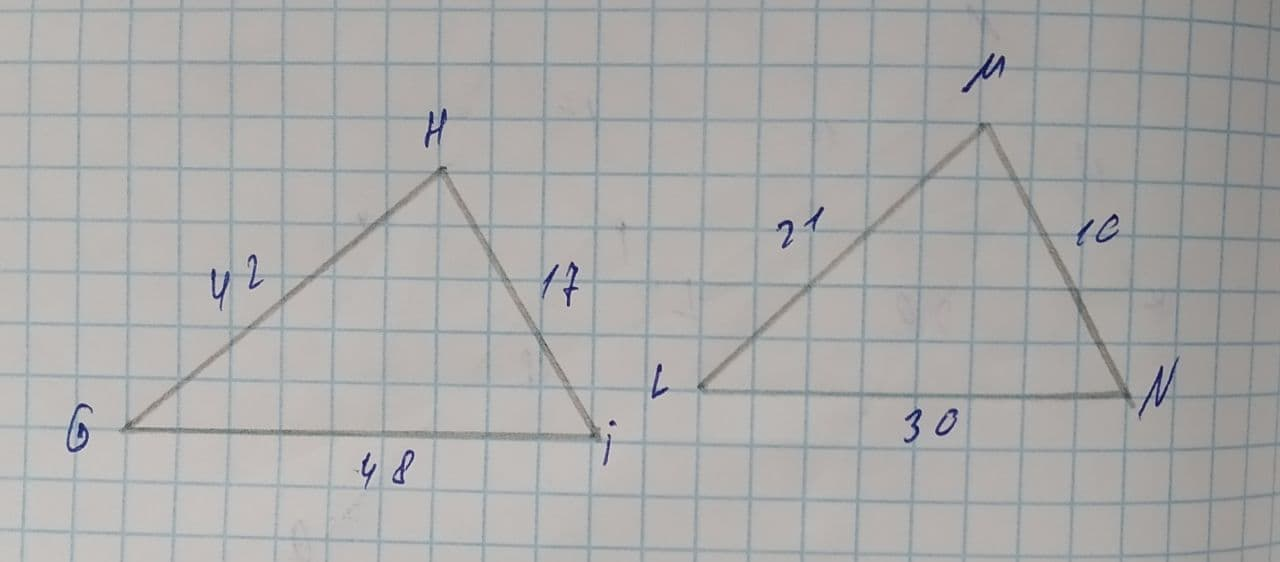# Determine (if possible) the similarity postulate that proves the following two triangles are similar:Dottie Parra 2021-08-05 Answered
Determine (if possible) the similarity postulate that proves the following two triangles are similar:AA similarity postulate
SAS similarity postulate
SSS similarity postulate
These triangles are not similar
You can still ask an expert for help

• Questions are typically answered in as fast as 30 minutes

Solve your problem for the price of one coffee

• Math expert for every subject
• Pay only if we can solve itinsonsipthinye
Step 1
Given two triangles are :Step 2
$\mathrm{△}GHI$,
GH=42, HI=17, IG=48
$\mathrm{△}LMN$,
LM=21, MN=10, NL=30
Step 3
We know that if two triangles are similar then their sides are in equal ratio.
if are similar then,
$\frac{GH}{LM}=\frac{HI}{MN}=\frac{GI}{LN}$
Here,
$\frac{GH}{LM}=\frac{42}{21}=2$
$\frac{HI}{MN}=\frac{17}{10}=1.7$
$\frac{GI}{LN}=\frac{48}{30}=1.6$,
That means, $\frac{GH}{LM}\ne q\frac{HI}{MN}\ne q\frac{GI}{LN}$
Hence given two triangles are not similar, $\mathrm{△}GHIn\sim \mathrm{△}LMN$
Answer : These triangles are not similar.